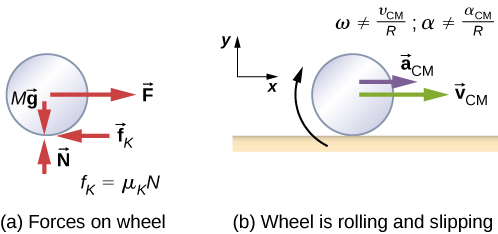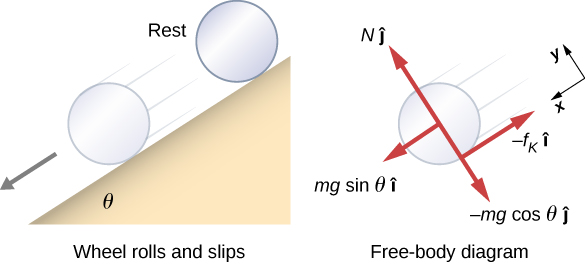# 11.1 Rolling motion  (Page 3/6)

 Page 3 / 6

## Significance

1. The linear acceleration is linearly proportional to $\text{sin}\phantom{\rule{0.2em}{0ex}}\theta .$ Thus, the greater the angle of the incline, the greater the linear acceleration, as would be expected. The angular acceleration, however, is linearly proportional to $\text{sin}\phantom{\rule{0.2em}{0ex}}\theta$ and inversely proportional to the radius of the cylinder. Thus, the larger the radius, the smaller the angular acceleration.
2. For no slipping to occur, the coefficient of static friction must be greater than or equal to $\left(1\text{/}3\right)\text{tan}\phantom{\rule{0.2em}{0ex}}\theta$ . Thus, the greater the angle of incline, the greater the coefficient of static friction must be to prevent the cylinder from slipping.

Check Your Understanding A hollow cylinder is on an incline at an angle of $60\text{°}.$ The coefficient of static friction on the surface is ${\mu }_{S}=0.6$ . (a) Does the cylinder roll without slipping? (b) Will a solid cylinder roll without slipping?

a. ${\mu }_{\text{S}}\ge \frac{\text{tan}\phantom{\rule{0.2em}{0ex}}\theta }{1+\left(m{r}^{2}\text{/}{I}_{\text{CM}}\right)}$ ; inserting the angle and noting that for a hollow cylinder ${I}_{\text{CM}}=m{r}^{2},$ we have ${\mu }_{\text{S}}\ge \frac{\text{tan}\phantom{\rule{0.2em}{0ex}}60\text{°}}{1+\left(m{r}^{2}\text{/}m{r}^{2}\right)}=\frac{1}{2}\text{tan}\phantom{\rule{0.2em}{0ex}}60\text{°}=0.87;$ we are given a value of 0.6 for the coefficient of static friction, which is less than 0.87, so the condition isn’t satisfied and the hollow cylinder will slip; b. The solid cylinder obeys the condition ${\mu }_{\text{S}}\ge \frac{1}{3}\text{tan}\phantom{\rule{0.2em}{0ex}}\theta =\frac{1}{3}\text{tan}\phantom{\rule{0.2em}{0ex}}60\text{°}=0.58.$ The value of 0.6 for ${\mu }_{\text{S}}$ satisfies this condition, so the solid cylinder will not slip.

It is worthwhile to repeat the equation derived in this example for the acceleration of an object rolling without slipping:

${a}_{\text{CM}}=\frac{mg\phantom{\rule{0.2em}{0ex}}\text{sin}\phantom{\rule{0.2em}{0ex}}\theta }{m+\left({I}_{\text{CM}}\text{/}{r}^{2}\right)}.$

This is a very useful equation for solving problems involving rolling without slipping. Note that the acceleration is less than that for an object sliding down a frictionless plane with no rotation. The acceleration will also be different for two rotating cylinders with different rotational inertias.

## Rolling motion with slipping

In the case of rolling motion with slipping, we must use the coefficient of kinetic friction, which gives rise to the kinetic friction force since static friction is not present. The situation is shown in [link] . In the case of slipping, ${v}_{\text{CM}}-R\omega \ne 0$ , because point P on the wheel is not at rest on the surface, and ${v}_{P}\ne 0$ . Thus, $\omega \ne \frac{{v}_{\text{CM}}}{R},\alpha \ne \frac{{a}_{\text{CM}}}{R}$ .(a) Kinetic friction arises between the wheel and the surface because the wheel is slipping. (b) The simple relationships between the linear and angular variables are no longer valid.

## Rolling down an inclined plane with slipping

A solid cylinder rolls down an inclined plane from rest and undergoes slipping ( [link] ). It has mass m and radius r . (a) What is its linear acceleration? (b) What is its angular acceleration about an axis through the center of mass?

## Strategy

Draw a sketch and free-body diagram showing the forces involved. The free-body diagram is similar to the no-slipping case except for the friction force, which is kinetic instead of static. Use Newton’s second law to solve for the acceleration in the x -direction. Use Newton’s second law of rotation to solve for the angular acceleration.

## SolutionA solid cylinder rolls down an inclined plane from rest and undergoes slipping. The coordinate system has x in the direction down the inclined plane and y upward perpendicular to the plane. The free-body diagram shows the normal force, kinetic friction force, and the components of the weight m g → .

The sum of the forces in the y -direction is zero, so the friction force is now ${f}_{\text{k}}={\mu }_{\text{k}}N={\mu }_{\text{k}}mg\text{cos}\phantom{\rule{0.2em}{0ex}}\theta .$

Newton’s second law in the x -direction becomes

$\sum {F}_{x}=m{a}_{x},$
$mg\phantom{\rule{0.2em}{0ex}}\text{sin}\phantom{\rule{0.2em}{0ex}}\theta -{\mu }_{\text{k}}mg\phantom{\rule{0.2em}{0ex}}\text{cos}\phantom{\rule{0.2em}{0ex}}\theta =m{\left({a}_{\text{CM}}\right)}_{x},$

or

${\left({a}_{\text{CM}}\right)}_{x}=g\left(\text{sin}\phantom{\rule{0.2em}{0ex}}\theta -{\mu }_{\text{K}}\phantom{\rule{0.2em}{0ex}}\text{cos}\phantom{\rule{0.2em}{0ex}}\theta \right).$

The friction force provides the only torque about the axis through the center of mass, so Newton’s second law of rotation becomes

$\sum {\tau }_{\text{CM}}={I}_{\text{CM}}\alpha ,$
${f}_{\text{k}}r={I}_{\text{CM}}\alpha =\frac{1}{2}m{r}^{2}\alpha .$

Solving for $\alpha$ , we have

$\alpha =\frac{2{f}_{\text{k}}}{mr}=\frac{2{\mu }_{\text{k}}g\phantom{\rule{0.2em}{0ex}}\text{cos}\phantom{\rule{0.2em}{0ex}}\theta }{r}.$

## Significance

We write the linear and angular accelerations in terms of the coefficient of kinetic friction. The linear acceleration is the same as that found for an object sliding down an inclined plane with kinetic friction. The angular acceleration about the axis of rotation is linearly proportional to the normal force, which depends on the cosine of the angle of inclination. As $\theta \to 90\text{°}$ , this force goes to zero, and, thus, the angular acceleration goes to zero.

what are the types of equilibrium
what is the formula for component vector
what is the area of a cylinder
what is chemical formular of calcium hexanitrato manganite 3
meaning of 2To equal to T1
9.6 all problems solution
what is the difference between variables and dimensions
how to convert m³
convert 35m³ to m³
Vandi
what is the reference frame of Kepler's motion of planet
if C is the vector sum of A and B, C=A+B, what must be true about the direction and magnitudes of A and B if C=A+B? what must be true about the direction and magnitudes of A and B if C=0?
For the second question either |A| and |B|= 0 or |A|=-|B|, If |A|= -|B| and |A|>0, then Phi[A,B]=180 Degree or π rad
Allthese
For the first question let |A| and |B| >O, then it is possible that |C|< |A| and |C|<|B| if Phi [A,B]<90 degree= |A| and |C|>|B|
Allthese
A correction to the first question If Phi[A,B] is within 90-270 degrees, then |C|> |A| and |C|>|B| define Phi[A,B] ≡ X, If 90 degree  ≤ X ≤ 270 degree (Phi[A,B] defined as X is within 90 to 270 degree including 90 degree and 270 degree) Then |C|> |A| and |C|> |B|
Allthese
state the properties of a base
state 3 properties of a base
State three properties of a base
mubarak
1.they are bitter 2.they conduct electricity 3.they are slippery or soapy
Akindele
a stone is projected from the top of the tower 24.5m high with velocity of 39.2m/s at an angle of 30° with horizontal compute time taken to hit he ground
it took a car driver 30mins to travel a distance of 50km.what is the average speed
Suppose the master cylinder in a hydraulic system is at a greater height than the cylinder it is controlling. Explain how this will affect the force produced at the cylinder that is being controlled.
Why is popo less than atmospheric? Why is popo greater than pipi?
Louise
The old rubber boot shown below has two leaks. To what maximum height can the water squirt from Leak 1? How does the velocity of water emerging from Leak 2 differ from that of Leak 1? Explain your responses in terms of energy.
Louise
David rolled down the window on his car while driving on the freeway. An empty plastic bag on the floor promptly flew out the window. Explain why.
the pressure differential exerted a force on the bag greater than the gravitational force holding it on the floor.
gtitboi

#### Get Jobilize Job Search Mobile App in your pocket Now!By OpenStaxBy RhodesBy OpenStaxBy Stephen VoronBy OpenStaxBy Sarah WarrenBy Dionne MahaffeyBy OpenStaxBy JavaChamp TeamBy Stephen Voron